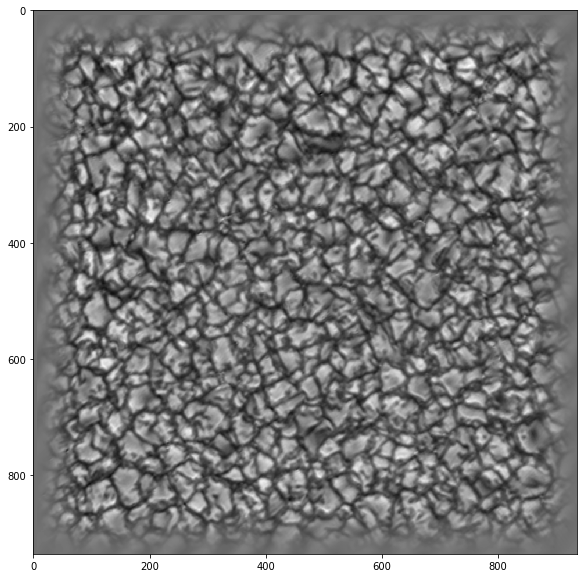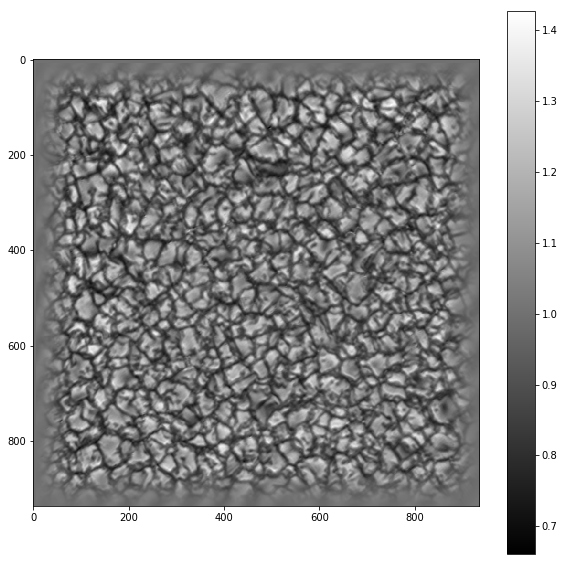# How to adjust a colorbar in Python

## Color bars in matplotlib¶

#### In this post I will show how to adjust the color bars to look nice next to an image.¶

In :
```## importing libraries
import pyfits
import matplotlib.pyplot as plt
import numpy as np
import pylab
```
In :
```## loading data with pyfits
p = '/home/fatima/Desktop/my_papers/paper_1/images/'
sufi_214 = pyfits.getdata(p+'flip_cont.fits')
fig = plt.figure(figsize=(10,10)) #defining the figure with the figure size (widthxheight)
ax.imshow(sufi_214,cmap='gray')
```
Out:
`<matplotlib.image.AxesImage at 0x7fb13cf12110>`#### If we want to add a colorbar, we can use the following:¶

In :
```from matplotlib import cm
plt.clf()
fig = plt.figure(figsize=(10,10))
im = ax.imshow(sufi_214,cmap='gray')
fig.colorbar(im,ax=ax,orientation='vertical')
#ax.set_aspect('auto')
```
Out:
`<matplotlib.colorbar.Colorbar at 0x7fb13d16a1d0>`
`<matplotlib.figure.Figure at 0x7fb13e006690>`#### As you can see, the length of the color bar exceeds that of the image, and it does not look very nice. The way to solve this is to divide the axis in which the image is plotted and assign a part of it to the colorbar.¶

In :
```from mpl_toolkits.axes_grid1 import make_axes_locatable, axes_size
```
In :
```plt.clf()
fig = plt.figure(figsize=(10,10))
`<matplotlib.colorbar.Colorbar at 0x7fb13cd1ed90>`
`<matplotlib.figure.Figure at 0x7fb13cd86a90>`